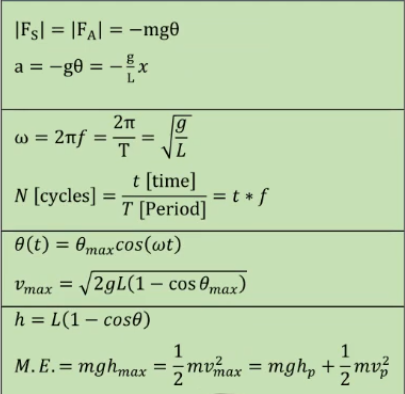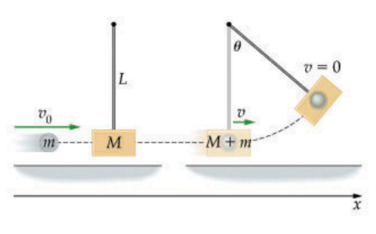# Problem: In a ballistic pendulum an object of mass m is fired with an initial speed v0 at a pendulum bob. The bob has a mass M, which is suspended by a rod of length L and negligible mass. After the collision, the pendulum and object stick together and swing to a maximum angular displacement θ as shown (Figure 1)Find an expression for v0, the initial speed of the fired object.Express your answer in terms of some or all of the variables m, M, L, θ and the acceleration due to gravity, g.

###### FREE Expert Solution

Momentum:

$\overline{){\mathbf{p}}{\mathbf{=}}{\mathbf{m}}{\mathbf{v}}}$

Kinetic energy:

$\overline{){\mathbf{K}}{\mathbf{=}}\frac{\mathbf{1}}{\mathbf{2}}{\mathbf{m}}{{\mathbf{v}}}^{{\mathbf{2}}}}$

Gravitational potential energy:

$\overline{){\mathbf{U}}{\mathbf{=}}{\mathbf{m}}{\mathbf{g}}{\mathbf{h}}}$

Pendulum SHM equations:91% (317 ratings)###### Problem Details

In a ballistic pendulum an object of mass m is fired with an initial speed v0 at a pendulum bob. The bob has a mass M, which is suspended by a rod of length L and negligible mass. After the collision, the pendulum and object stick together and swing to a maximum angular displacement θ as shown (Figure 1)Find an expression for v0, the initial speed of the fired object.

Express your answer in terms of some or all of the variables m, M, L, θ and the acceleration due to gravity, g.